Dr. Emmanuel Tsukerman

# Explore Python Libraries: Imbalanced-learn

• Jun 17, 2020
• 2,702 Views
• Jun 17, 2020
• 2,702 Views
Data
Data Analytics
Machine Learning
Python

## Introduction

As a data scientist working with real world data, at some point in your career you will face the challenge of constructing a machine learning model on imbalanced data. You will need savvy decision making on a number of questions, from selecting the correct metric to ensuring sufficient training of your model on the minor classes. To help you overcome these challenges to constructing an accurate and informative model, this guide will introduce you to the imbalanced-learn library and show you its top three most popular use cases.

## Overview of the Imbalanced-learn Library

One of the most promising approaches for tackling imbalanced data is via resampling techniques. Conveniently, the Python imbalanced-learn library offers a great number of resampling techniques and implementations, including the three most useful: SMOTE, ADASYN, and Balanced Random Forest.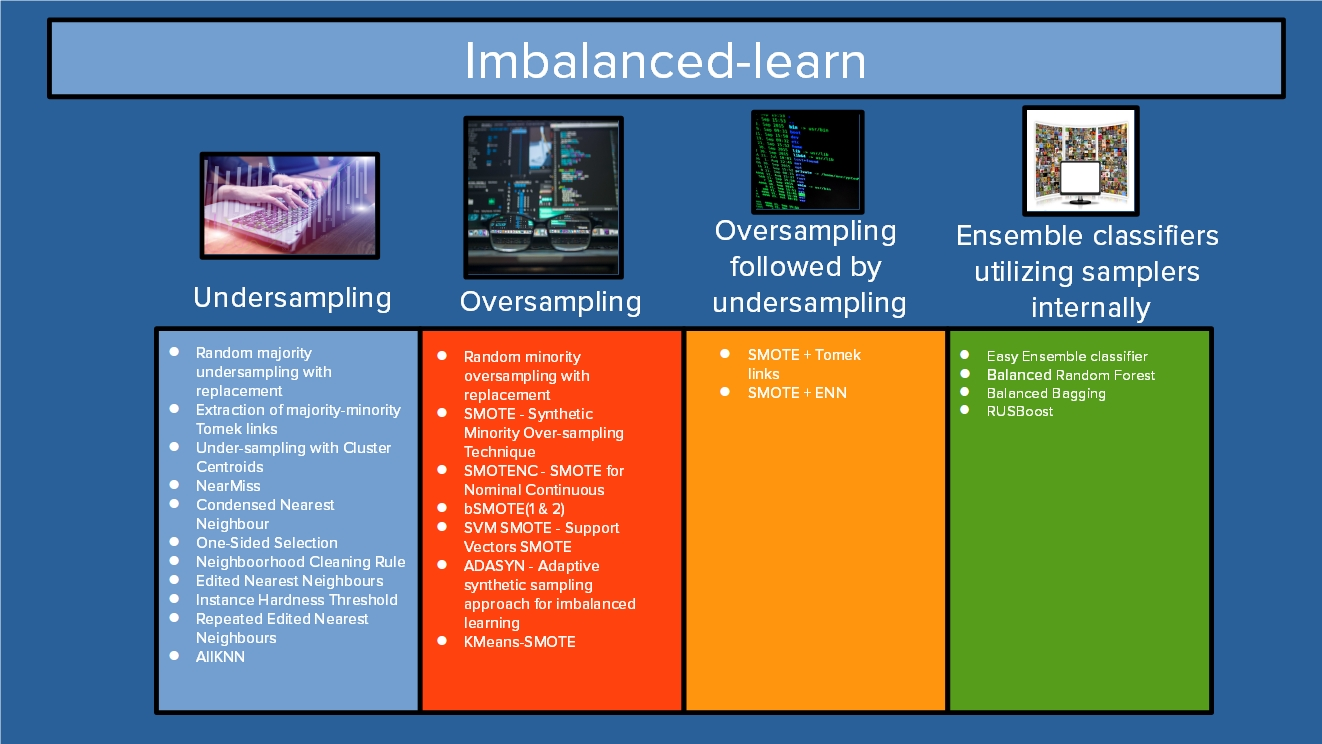Getting started with imbalanced-learn is not difficult, and the package is easy to understand even for data scientists in the first few years of their careers.

## Installation

Simply run:

``1pip install -U imbalanced-learn``
bash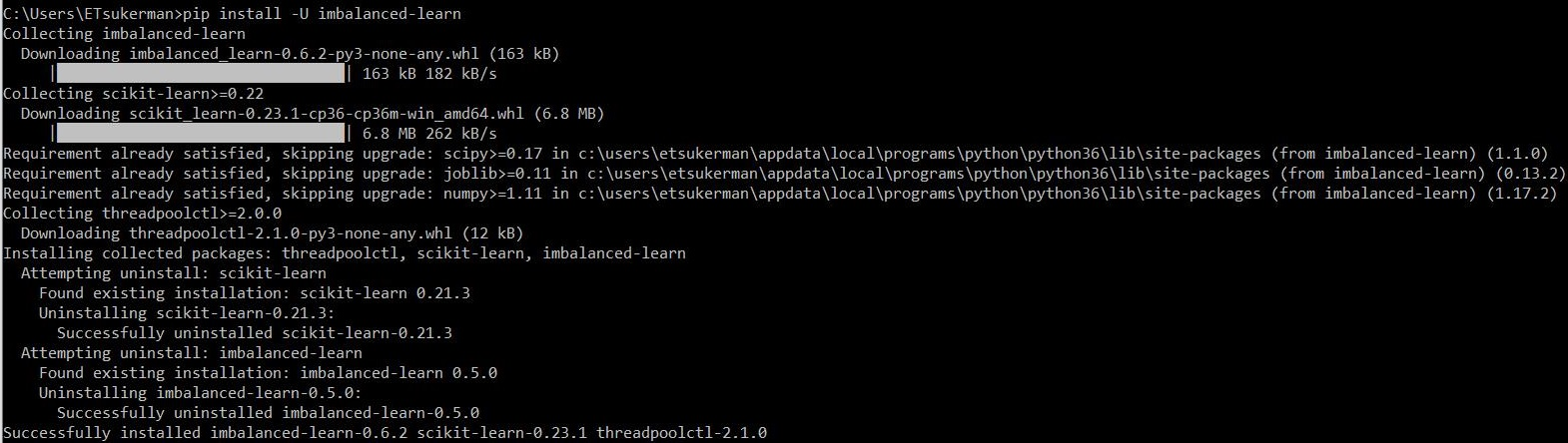That’s it! Imbalanced-learn is installed and ready for use.

For the remainder of the guide, code is available at https://github.com/emmanueltsukerman/imbalanced-learn-tutorial.

## Use Case 1: SMOTE

SMOTE stands for “Synthetic Minority Oversampling Technique” and is one of the most commonly utilized resampling techniques. At a high level, to oversample, pick a sample from the minority class (call it S), and then pick one of its neighbors, N. Then pick a random point on the line segment between S and N. This random new point is the synthetic sample you have created using SMOTE. Repeating this over and over you can create more and more new samples to even out your class imbalance.

Now you’ll see how to use imbalanced-learn’s implementation of SMOTE. To illustrate, you will use the Credit Card Fraud Detection dataset, available at https://www.kaggle.com/mlg-ulb/creditcardfraud.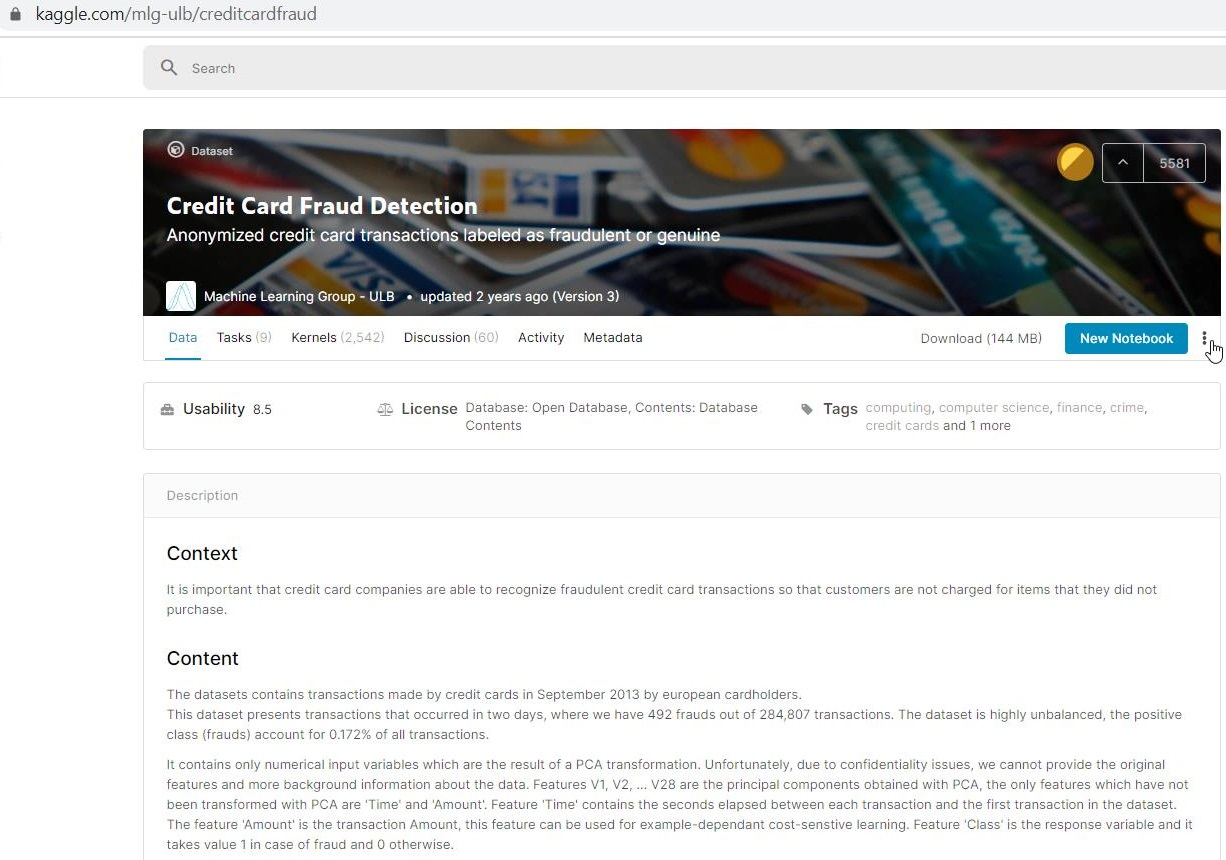This dataset is highly imbalanced, so it will serve as a great demo set.

Once you have downloaded the dataset, you can see that it consists of 28 anonymized features, as well as time, amount, and class.

``````1import pandas as pd
2
python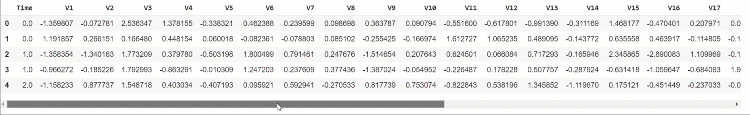Place the features into an array `X` and the labels into an array `y`.

``````1X = df.drop('Class', axis=1)
2y = df['Class']``````
python

You will now oversample the minor class via SMOTE so that the two classes in the dataset are balanced.

``````1from imblearn.over_sampling import SMOTE
2
3X_smote, y_smote = SMOTE().fit_sample(X, y)``````
python

You can see that the classes are now balanced:

``````1X_smote = pd.DataFrame(X_smote)
2y_smote = pd.DataFrame(y_smote)
3y_smote.iloc[:, 0].value_counts()``````
python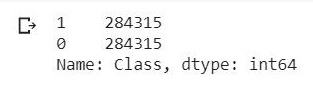Create a train-test split:

``````1from sklearn.model_selection import train_test_split
2
3X_train, X_test, y_train, y_test = train_test_split(
4    X_smote, y_smote, test_size=0.2, random_state=0
5)
6import numpy as np
7
8X_train = np.array(X_train)
9X_test = np.array(X_test)
10y_train = np.array(y_train)
11y_test = np.array(y_test)``````
python

Then train and test a simple classifier:

``````1from sklearn import tree
2
3clf = tree.DecisionTreeClassifier()
4clf.fit(X_train, y_train)``````
python

Finally, see the performance:

``````1from sklearn.metrics import confusion_matrix
2import seaborn as sns
3
4y_pred = clf.predict(X_test)
5cm2 = confusion_matrix(y_test, y_pred.round())
6sns.heatmap(cm2, annot=True, fmt=".0f")``````
python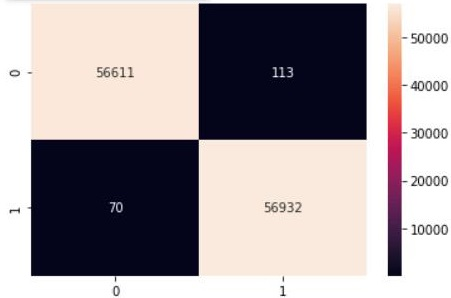As you can see, by utilizing SMOTE we have ensured that the classifier produces relatively balanced classifications, as opposed to an unsuccessful model, in which the classifier might classify all samples as belonging to the majority class.

ADASYN takes ideas from SMOTE and builds on them. In particular, ADASYN selects minority samples S so that “more difficult to classify” minority samples are more likely to be selected. This allows the classifier to have more opportunity to learn tough instances. The code for ADASYN is entirely analogous to that of SMOTE, except you just replace the word “SMOTE” with “ADASYN”.

``````1from imblearn.over_sampling import ADASYN
2
4<snip>
5from sklearn.metrics import confusion_matrix
6import seaborn as sns
7
8y_pred = clf.predict(X_test)
9cm2 = confusion_matrix(y_test, y_pred.round())
10sns.heatmap(cm2, annot=True, fmt=".0f")``````
python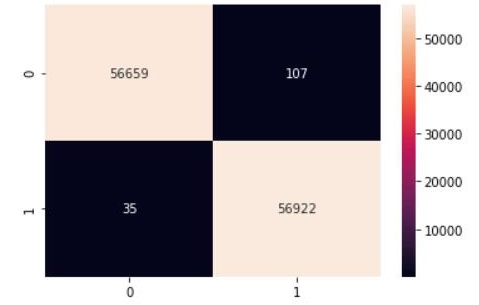## Use Case 3: Balanced Random Forest

`BalancedRandomForestClassifier` differs from SMOTE and ADASYN in that it is not a resampling method, but rather a classifier in which the training employs resampling internally. For more info, see Breinman et al., Using Random Forest to Learn Imbalanced Data.

Instantiate the classifier:

``````1from imblearn.ensemble import BalancedRandomForestClassifier
2
3brf = BalancedRandomForestClassifier(n_estimators=100, random_state=0)``````
python

Then split the dataset into training and testing:

``1X_train, X_test, y_train, y_test = train_test_split(X, y, test_size=0.2, random_state=0)``
python

Then fit and predict:

``````1brf.fit(X_train, y_train)
2y_pred = brf.predict(X_test)
3cm2 = confusion_matrix(y_test, y_pred.round())
4sns.heatmap(cm2, annot=True, fmt=".0f")``````
python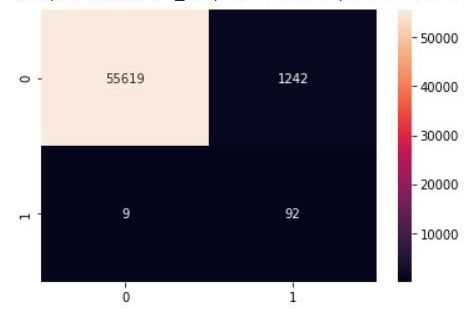## Conclusion

It’s a great skill for a data scientist to be able to confidently tackle problems with imbalanced data. The library imbalanced-learn supplies you with just the right tools to do so. To delve deeper into the library, I recommend the well-written official documentation for imbalanced-learn available at https://imbalanced-learn.readthedocs.io/en/stable/. Happy learning!